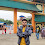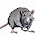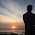# HackerRank Java Date and Time problem solution

In this HackerRank Java Date and Time problem in a java programming language, You are given a date. You just need to write the method, getDay, which returns the day on that date.

## HackerRank Java Date and Time problem solution.

```import java.io.*;
import java.math.*;
import java.security.*;
import java.text.*;
import java.util.*;
import java.util.concurrent.*;
import java.util.regex.*;

class Result {

/*
* Complete the 'findDay' function below.
*
* The function is expected to return a STRING.
* The function accepts following parameters:
*  1. INTEGER month
*  2. INTEGER day
*  3. INTEGER year
*/

public static String findDay(int month, int day, int year) {

Calendar cal = Calendar.getInstance();
cal.set(Calendar.MONTH,month-1);
cal.set(Calendar.DAY_OF_MONTH,day);
cal.set(Calendar.YEAR,year);
String dayOfWeek = cal.getDisplayName(Calendar.DAY_OF_WEEK, Calendar.LONG, Locale.US).toUpperCase();
return dayOfWeek;

}

}

public class Solution {
public static void main(String[] args) throws IOException {
BufferedWriter bufferedWriter = new BufferedWriter(new FileWriter(System.getenv("OUTPUT_PATH")));

int month = Integer.parseInt(firstMultipleInput);

int day = Integer.parseInt(firstMultipleInput);

int year = Integer.parseInt(firstMultipleInput);

String res = Result.findDay(month, day, year);

bufferedWriter.write(res);
bufferedWriter.newLine();

bufferedWriter.close();
}
}

```

## Second solution in java8 programming.

```import java.io.*;
import java.util.*;
import java.text.*;
import java.math.*;
import java.util.regex.*;

public class Solution {

public static void main(String[] args) {
try (Scanner scanner = new Scanner(System.in)) {
int month = scanner.nextInt();
int day = scanner.nextInt();
int year = scanner.nextInt();

Calendar calendar = Calendar.getInstance();
calendar.set(Calendar.DATE, day);
calendar.set(Calendar.MONTH, month - 1);
calendar.set(Calendar.YEAR, year);

System.out.println(calendar.getDisplayName(Calendar.DAY_OF_WEEK,
Calendar.LONG, new Locale("en", "US")).toUpperCase());
}
}
}```

### Third solution

```import java.util.Arrays;
import java.util.Calendar;
import java.util.List;
import java.util.Scanner;

static List<String> days = Arrays.asList("SUNDAY", "MONDAY", "TUESDAY", "WEDNESDAY", "THURSDAY", "FRIDAY", "SATURDAY");

public static String getDay(String day, String month, String year) {

int y = Integer.parseInt(year);
int m = Integer.parseInt(month);
int d = Integer.parseInt(day);

Calendar c = Calendar.getInstance();
c.set(y, m-1, d);

int p = c.get(Calendar.DAY_OF_WEEK);
String s = days.get(p-1);
return s;
}

public static void main(String[] args) {
Scanner in = new Scanner(System.in);
String month = in.next();
String day = in.next();
String year = in.next();

System.out.println(getDay(day, month, year));

}
}```

1.Hello Yash bro...!
//here
cal.set(Calendar.MONTH,month-1);// at this line why u kept "-1" please tell me for it.

1.because in java january is start from 0.

2.3.2.Hello Yash, why Calendar.LONG and Locale.US are taken? please explain me

3.Anyone pleae tell me why they have put Calendar.LONG and Locale.US in the program?

1.Calendar.LONG is used to output January instead of jan and Locale.US helps us to specify the time ig

4.This is an excellent post - thank you for sharing it! I always relish the opportunity to read such superb content filled with valuable information. The ideas presented here are truly best and exceptionally captivating, making the post thoroughly enjoyable. Please continue with your fantastic work.
Unveiling the Power of Java Streams API